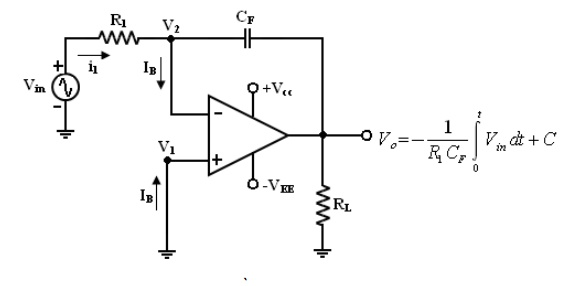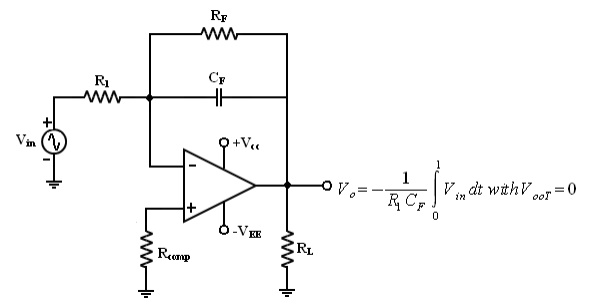# Integrator

A circuit in which the output voltage waveform is the integral of the input voltage waveform is the integrator or Integration Amplifier.

Integrator:

A circuit in which the output voltage waveform is the integral of the input voltage waveform is the integrator or Integration Amplifier. Such a circuit is obtained by using a basic inverting amplifier configuration if the feedback resistor RF is replaced by a capacitor CF.

The expression for the output voltage V0 can be obtained by KVL eqn at node V2.where C integration constant A

eqn (3) indicates that the output is directly proportional to the negative integral of the input volts and inversely proportional to the time constant R1 CF .

Ex: If the input is sine wave -> output is cosine wave.

If the input is square wave -> output is triangular wave.These waveform with assumption of R1Cf = 1, Vout =0V (i.e) C =0.

When Vin = 0 the integrator works as an open loop amplifier because the capacitor CF acts an open circuit to the input offset voltage Vio.

Or

The Input offset voltage Vio and the part of the input are charging capacitor CF produce the error voltage at the output of the integrator.

Practical Integrator:Practical Integrator to reduce the error voltage at the output, a resistor RF is connected across the feedback capacitor CF.

Thus RF limits the low frequency gain and hence minimizes the variations in the output voltages. The frequency response of the basic integrator, shown from this fb is the frequency at which the gain is dB and is given by,

Both the stability and low frequency roll-off problems can be corrected by the addition of a resistor RF in the practical integrator.

Stability -> refers to a constant gain as frequency of an input signal is varied over a certain range.

Low frequency -> refers to the rate of decrease in gain roll off at lower frequencies.

From the fig of practical Integrators,

f is some relative operating frequency and for frequencies f to fa to gain RF / R1 is constant. After fa the gain decreases at a rate of 20dB/decade or between fa and fb the circuit act as an integrator.

Generally the value of fa and in turn R1 CF and RF CF values should be selected such that fa<fb. In fact, the input signal will be integrated properly if the time period T of the signal is larger than

or

equal to RF CF, (i.e) T >= RF CF @@@@ 6

Uses:

Most commonly used in analog computers.